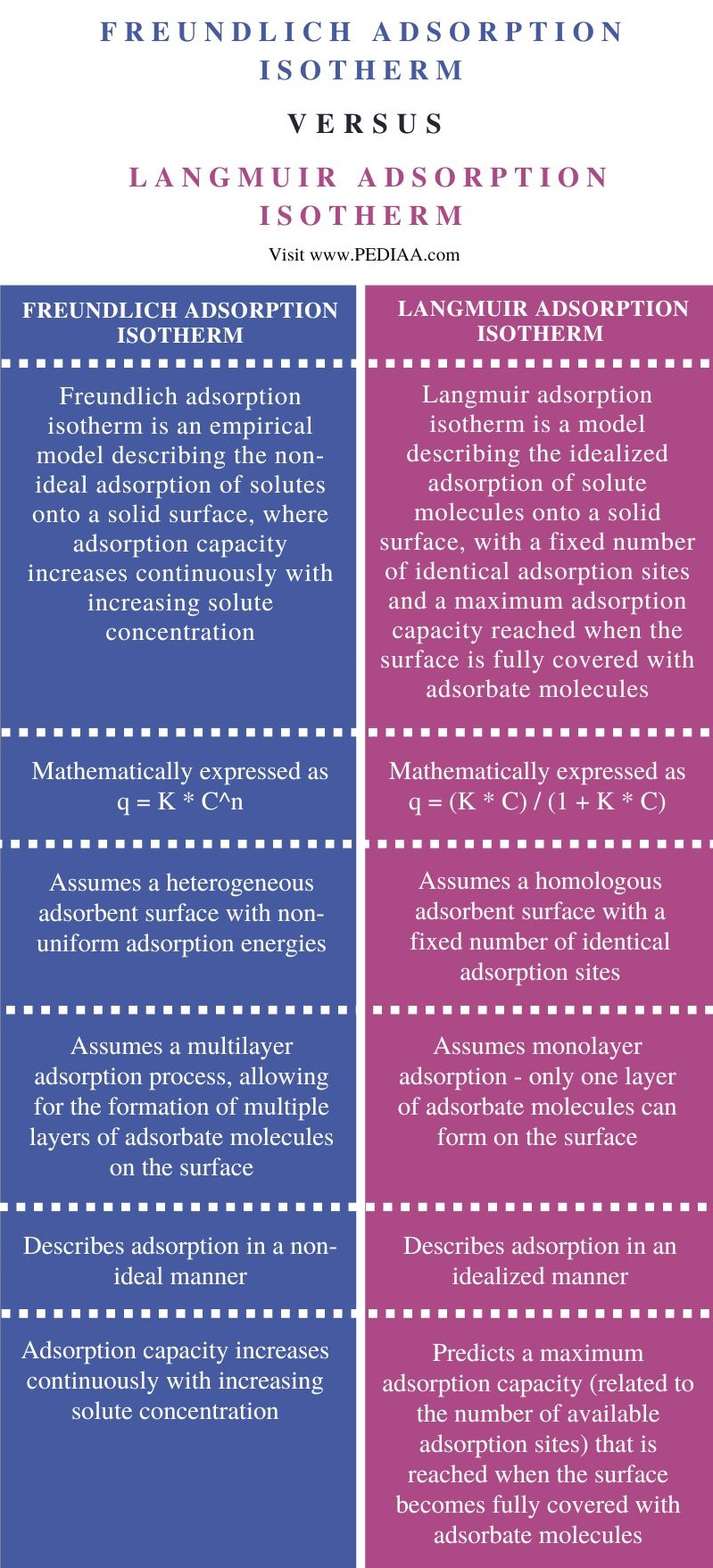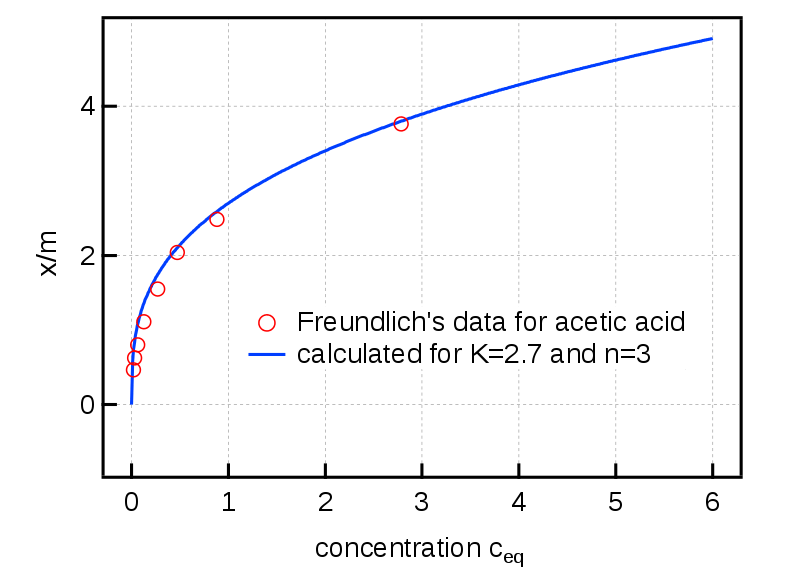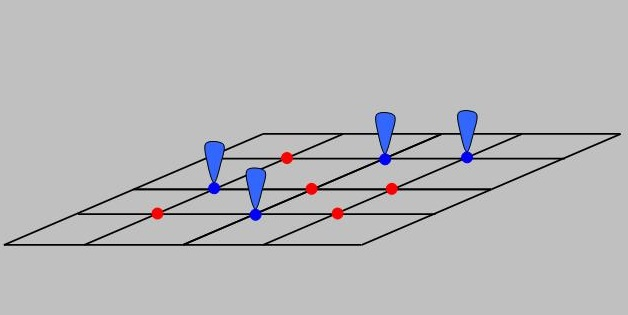# What is the Difference Between Freundlich and Langmuir Adsorption Isotherm

The main difference between Freundlich and Langmuir adsorption isotherm is that Freundlich isotherm is more flexible, allowing for multilayer adsorption and non-uniform surfaces. In contrast, Langmuir isotherm assumes monolayer adsorption on a homogeneous surface with a fixed number of sites.

Freundlich and Langmuir adsorption isotherms are mathematical models used to describe the adsorption behavior of solutes onto a solid surface. While they both indicate the solute concentration in the solution to the amount adsorbed onto the surface, there are several differences between Freundlich and Langmuir adsorption isotherms.

### Key Areas Covered

1. What is Freundlich Adsorption Isotherm
2. What is Langmuir Adsorption Isotherm
3. Similarities Between Freundlich and Langmuir Adsorption Isotherm
– Outline of Common Features
4. Difference Between Freundlich and Langmuir Adsorption Isotherm
– Comparison of Key Differences

### Key Terms## What is Freundlich Adsorption Isotherm

The Freundlich adsorption isotherm is an empirical model that describes the adsorption of solutes onto a solid surface. It is named after the German chemist Herbert Freundlich, who introduced this concept in 1906. The Freundlich isotherm is widely used in surface chemistry and is crucial in understanding and predicting adsorption phenomena.

The Freundlich isotherm applies to systems with heterogeneous surfaces and assumes that the adsorption occurs on multiple sites with varying affinities. It suggests that the adsorbate molecules interact with the adsorbent surface through weak forces, such as van der Waals interactions or hydrogen bonding. These interactions can occur on different sites, each having a unique affinity for the solute molecules.

### Calculation of Freundlich Isotherm

Mathematically, the Freundlich isotherm equation is expressed as:

q = K * C^n

Where q is the amount of solute adsorbed per unit mass of adsorbent, C is the equilibrium concentration of the solute in the solution, K is the Freundlich constant related to the adsorption capacity, and n is the Freundlich exponent related to the adsorption intensity or nonlinearity of the process.

The Freundlich constant (K) is a measure of the adsorption capacity of the adsorbent. It represents the maximum amount of solute that can be adsorbed per unit mass of adsorbent at a specific concentration. The value of K depends on factors such as the nature of the adsorbent, the adsorbate, and the temperature. A higher value of K indicates a higher adsorption capacity.

The Freundlich exponent (n) indicates the adsorption intensity or nonlinearity of the process. A value of n greater than 1 suggests favorable adsorption, meaning that as the solute concentration increases, the amount adsorbed also increases at an increasing rate. A value of n less than 1 suggests less favorable adsorption, where the amount adsorbed increases at a decreasing rate as the solute concentration increases. Furthermore, a value of n equal to 1 indicates a linear relationship between adsorbate concentration and adsorbed amount.

The Freundlich isotherm equation can be transformed into a linear form by taking the logarithm of both sides:

log(q) = log(K) + (n * log(C))

This linear form allows for determining the Freundlich constant (K) and the Freundlich exponent (n) through a linear regression analysis of experimental data.### Limitations of Freundlich Isotherm

However, it is important to note that the Freundlich isotherm has certain limitations. It assumes that the adsorption occurs on a heterogeneous surface with multiple sites. Still, it does not provide information about the specific nature of these sites or the interactions occurring at each site. The model also does not consider surface saturation or the possibility of multilayer adsorption. Furthermore, the Freundlich isotherm may not apply to systems where the solute concentration is very high or where there is a strong interaction between the solute molecules.

The Freundlich isotherm is widely used in various fields, including environmental science, catalysis, separation processes, and drug delivery. It provides valuable insights into the adsorption behavior of solutes onto different adsorbents and helps understand factors influencing adsorption, such as surface area, pore size, temperature, and solution composition.

## What is Langmuir Adsorption Isotherm

### Calculation of Langmuir Adsorption Isotherm

Mathematically, the Langmuir isotherm equation is expressed as:

q = (K * C) / (1 + K * C)

Where q is the amount of solute adsorbed per unit mass of adsorbent, C is the equilibrium concentration of the solute in the solution, and K is the Langmuir constant related to the adsorption equilibrium constant.

The Langmuir constant (K) is a measure of the affinity of the solute for the adsorbent surface. It represents the ratio of the adsorption rate constant to the desorption rate constant and provides information about the strength of the solute-adsorbent interaction. A higher value of K indicates a stronger interaction and a higher affinity of the solute for the adsorbent surface.

The Langmuir isotherm equation can be transformed into a linear form by taking the reciprocal of both sides:

1/q = (1/K) * (1/C) + (1/K)

This linear form allows for determining the Langmuir constant (K) through a linear regression analysis of experimental data.Figure 2: Langmuir Adsorption Model [Schematic of occupied (blue) and unoccupied (red) equivalent sites, demonstrating model assumptions: unit occupancy of adsorption sites (heavy dots) and immobility of adsorbates]

### Applications of Langmuir Isotherm

The Langmuir isotherm has several important implications and applications. It allows for calculating the maximum adsorption capacity of the adsorbent, represented by the Langmuir constant (K). The maximum adsorption capacity corresponds to the amount of solute that can be adsorbed when all adsorption sites on the surface are occupied.

## Similarities Between Freundlich and Langmuir Adsorption Isotherm

• Freundlich and Langmuir isotherms describe the relationship between the adsorbate concentration in the solution and the amount of adsorbate adsorbed onto the adsorbent surface.
• Both isotherms can describe adsorption at equilibrium, where the adsorption rate is equal to the desorption rate.
• They are empirical models, meaning they are based on experimental observations and do not have a rigorous theoretical basis.

## Difference Between Freundlich and Langmuir Adsorption Isotherm

### Definition

Freundlich adsorption isotherm is an empirical model describing the non-ideal adsorption of solutes onto a solid surface, where adsorption capacity increases continuously with increasing solute concentration. In contrast, Langmuir adsorption isotherm is a model describing the idealized adsorption of solute molecules onto a solid surface, with a fixed number of identical adsorption sites and a maximum adsorption capacity reached when the surface is fully covered with adsorbate molecules.

### Mathematical Expression

The Freundlich isotherm equation is mathematically expressed as q = K * C^n, while the Langmuir isotherm equation is expressed as q = (K * C) / (1 + K * C).

### Assumptions

Freundlich isotherm assumes a heterogeneous adsorbent surface with non-uniform adsorption energies. It also assumes a multilayer adsorption process, allowing for the formation of multiple layers of adsorbate molecules on the surface. On the other hand, Langmuir isotherm assumes a homologous adsorbent surface with a fixed number of identical adsorption sites. It assumes monolayer adsorption, meaning only one layer of adsorbate molecules can form on the surface.

Moreover, Freundlich isotherm describes adsorption in a non-ideal manner, where the adsorption capacity increases continuously with increasing solute concentration. The Freundlich exponent (n) indicates the adsorption intensity or favorability. Langmuir isotherm describes adsorption in an idealized manner, predicting a maximum adsorption capacity (related to the number of available adsorption sites) reached when the surface becomes fully covered with adsorbate molecules. It assumes no further adsorption occurs beyond this point.

## Conclusion

Freundlich and Langmuir adsorption isotherms are mathematical models that describe the adsorption behavior of solutes onto a solid surface. Freundlich isotherm allows for multilayer adsorption and non-uniform surfaces. In contrast, Langmuir isotherm assumes monolayer adsorption on a homogeneous surface with a fixed number of sites. Thus, this is the main difference between Freundlich and Langmuir adsorption isotherm.

##### Reference:

1. “Freundlich Equation – An Overview.” Science Direct.
2. “Langmuir Isotherm – An Overview.” Science Direct.

Image Courtesy:

1. “Freundlich acetic acid” By Toshiyouri – Own work(CC BY-SA 4.0) via Commons Wikimedia
2. “Langmuir Adsorption Model” By SmugBoy – Own work (CC BY-SA 3.0) via Commons Wikimedia